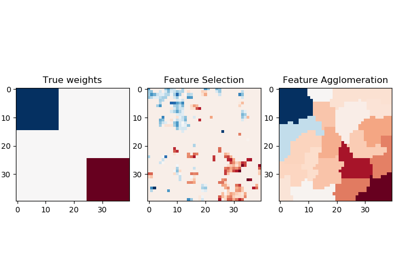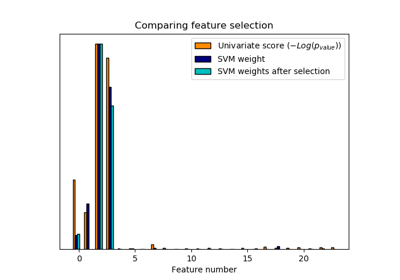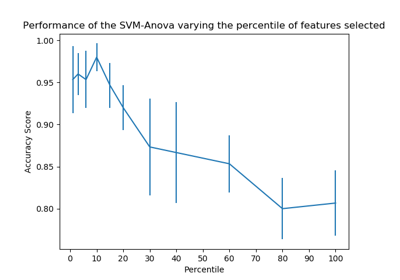# sklearn.feature_selection.SelectPercentile¶

class sklearn.feature_selection.SelectPercentile(score_func=<function f_classif>, percentile=10)[source]

Select features according to a percentile of the highest scores.

Read more in the User Guide.

Parameters: score_func : callable Function taking two arrays X and y, and returning a pair of arrays (scores, pvalues) or a single array with scores. Default is f_classif (see below “See also”). The default function only works with classification tasks. percentile : int, optional, default=10 Percent of features to keep. scores_ : array-like, shape=(n_features,) Scores of features. pvalues_ : array-like, shape=(n_features,) p-values of feature scores, None if score_func returned only scores.

f_classif
ANOVA F-value between label/feature for classification tasks.
mutual_info_classif
Mutual information for a discrete target.
chi2
Chi-squared stats of non-negative features for classification tasks.
f_regression
F-value between label/feature for regression tasks.
mutual_info_regression
Mutual information for a continuous target.
SelectKBest
Select features based on the k highest scores.
SelectFpr
Select features based on a false positive rate test.
SelectFdr
Select features based on an estimated false discovery rate.
SelectFwe
Select features based on family-wise error rate.
GenericUnivariateSelect
Univariate feature selector with configurable mode.

Notes

Ties between features with equal scores will be broken in an unspecified way.

Examples

>>> from sklearn.datasets import load_digits
>>> from sklearn.feature_selection import SelectPercentile, chi2
>>> X.shape
(1797, 64)
>>> X_new = SelectPercentile(chi2, percentile=10).fit_transform(X, y)
>>> X_new.shape
(1797, 7)


Methods

 fit(self, X, y) Run score function on (X, y) and get the appropriate features. fit_transform(self, X[, y]) Fit to data, then transform it. get_params(self[, deep]) Get parameters for this estimator. get_support(self[, indices]) Get a mask, or integer index, of the features selected inverse_transform(self, X) Reverse the transformation operation set_params(self, \*\*params) Set the parameters of this estimator. transform(self, X) Reduce X to the selected features.
__init__(self, score_func=<function f_classif at 0x7efe30bb2158>, percentile=10)[source]
fit(self, X, y)[source]

Run score function on (X, y) and get the appropriate features.

Parameters: X : array-like, shape = [n_samples, n_features] The training input samples. y : array-like, shape = [n_samples] The target values (class labels in classification, real numbers in regression). self : object
fit_transform(self, X, y=None, **fit_params)[source]

Fit to data, then transform it.

Fits transformer to X and y with optional parameters fit_params and returns a transformed version of X.

Parameters: X : numpy array of shape [n_samples, n_features] Training set. y : numpy array of shape [n_samples] Target values. X_new : numpy array of shape [n_samples, n_features_new] Transformed array.
get_params(self, deep=True)[source]

Get parameters for this estimator.

Parameters: deep : boolean, optional If True, will return the parameters for this estimator and contained subobjects that are estimators. params : mapping of string to any Parameter names mapped to their values.
get_support(self, indices=False)[source]

Get a mask, or integer index, of the features selected

Parameters: indices : boolean (default False) If True, the return value will be an array of integers, rather than a boolean mask. support : array An index that selects the retained features from a feature vector. If indices is False, this is a boolean array of shape [# input features], in which an element is True iff its corresponding feature is selected for retention. If indices is True, this is an integer array of shape [# output features] whose values are indices into the input feature vector.
inverse_transform(self, X)[source]

Reverse the transformation operation

Parameters: X : array of shape [n_samples, n_selected_features] The input samples. X_r : array of shape [n_samples, n_original_features] X with columns of zeros inserted where features would have been removed by transform.
set_params(self, **params)[source]

Set the parameters of this estimator.

The method works on simple estimators as well as on nested objects (such as pipelines). The latter have parameters of the form <component>__<parameter> so that it’s possible to update each component of a nested object.

Returns: self
transform(self, X)[source]

Reduce X to the selected features.

Parameters: X : array of shape [n_samples, n_features] The input samples. X_r : array of shape [n_samples, n_selected_features] The input samples with only the selected features.

## Examples using sklearn.feature_selection.SelectPercentile¶Feature agglomeration vs. univariate selectionUnivariate Feature SelectionSVM-Anova: SVM with univariate feature selection